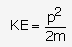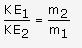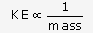# Define kinetic energy. Derive an expression for the kinetic energy

Define kinetic energy. Derive an expression for the kinetic energy possessed by an object of mass ‘m’ moving with a velocity ‘v’.

A light and a heavy object have the same momentum. Find the ratio of their kinetic energies. Which one has a larger kinetic energy?

Energy possessed by a body by virtue of its motion is kinetic energy.

Derivation:
Consider a body of mass m initially at rest. If on application of force F, it travels a distance s, then

Work done, W = F x s
By Newton’s second law, F = ma
W = ma x s
where, a = acceleration
Using equation of motion v2 - u2 =2as, we get
(v2-u2)/2 = as
W = m x (v2 - u2)/2
As intial velocity is zero, W = ½mv2
This work done is stored as kinetic energy of the object.

So, KE = ½ mv2
Let the mass of lighter object be m1 and that of heavier object be m2.
m1< m2
p1= p2For a given momentum, KE∝ 1/m SoSince, so lighter object has larger kinetic energy.